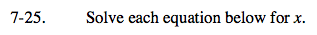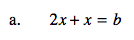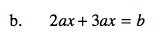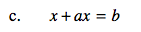### Home > A2C > Chapter 7 > Lesson 7.1.2 > Problem7-25

7-25.Combine like terms.

$\textit{x}=\frac{\textit{b}}{3}$Combine like terms. Then divide both sides by 5a to solve for x.Factor out an x on the left side. Solve for x by dividing.

$\textit{x}=\frac{\textit{b}}{1+\textit{a}}$